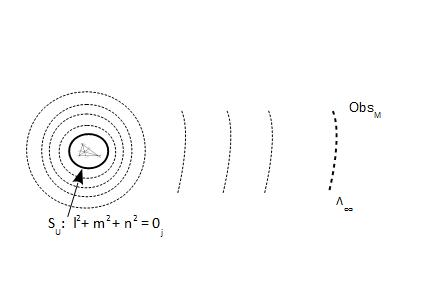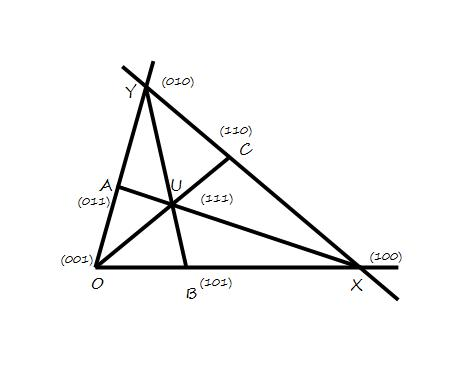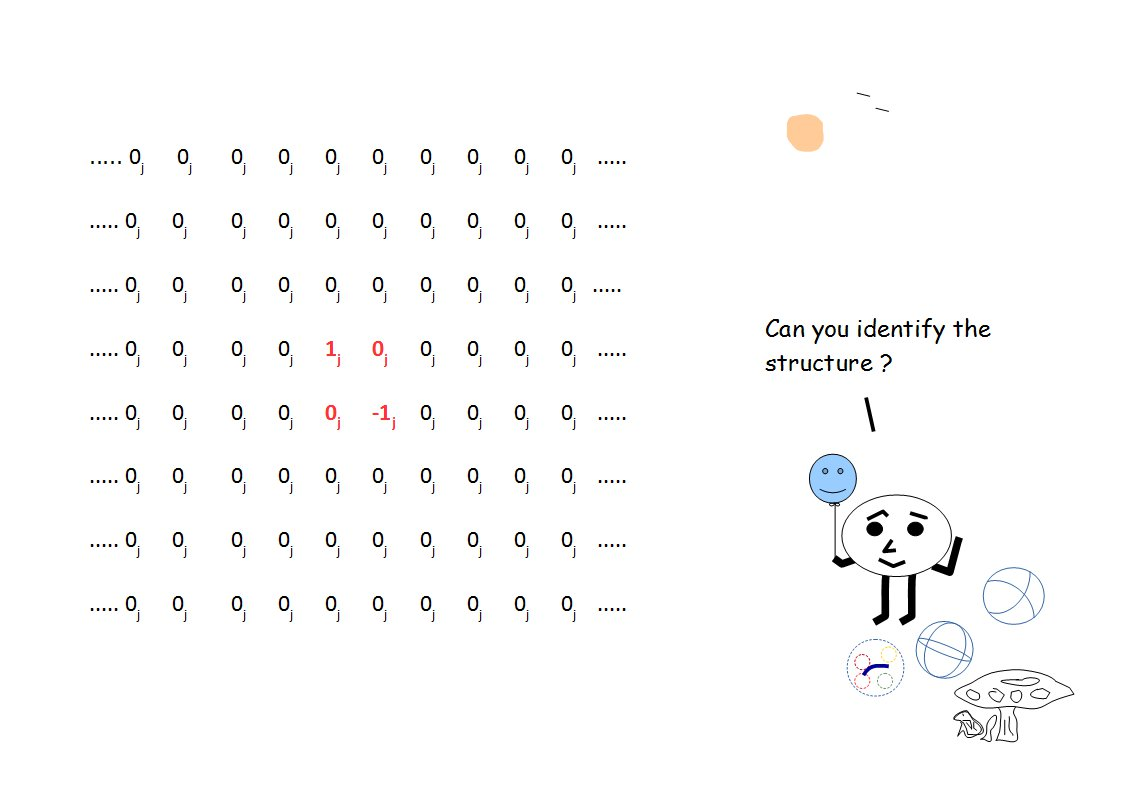# Home

 σz   and I 11th October 2015 " When you observe an interesting property of numbers, ask if perhaps you are not seeing, in the 1 x 1 case, an interesting property of matrices. " - Olga Taussky                 " I wrote this book for myself. I wanted to piece together carefully my own path through Galois Theory, a subject whose mathematical centrality and beauty I had often glimpsed, but one which I had never properly organized in my own mind." - Charles R. Hadlock, “Field Theory and its Classical Problems”. We have developed a rather important geometrical structure with central-force nature, with associated 4π-symmetry.  The physical description corresponding to SU was measured on Λ∞ plane by what we call the macroscopic observer ObsM.  This physical description as measured by ObsM, could have been of any dimensions except 0 or 1, but the underlying measurement-framework would not change.  The measured physical description must follow the constraints of the framework.     The important characteristics of the measurement-framework in the case under consideration, were the shell-structure and the central-force laws.  It should be clarified that the shell structure shown in Figure-1, was not a property of the the anharmonic coordinate representation.  Instead it was the consequence of how we applied a measurement scheme, in this case spin matrices σx ,  σy ,  and σz, to the anharmonic coordinates and then developed the physical description based on z-axis measurements.  The discrete nature of the structure was due to the constructibility constraints imposed upon the coefficients l, m, and n, i.e. theses coefficients must be rational.Figure-1: Anharmonic Coordinates + Spin Matrices -> Shell Structure. The σz matrix is given as follows,Some of the properties of zero-trace matrices such as the matrix σz , are as follows 1: 1. Given Σ λi = 0 s.t. |λi| = 1 for every i = 1, 2, .............., n; the eigenvalues are the end-point of a diameter for n = 2. 2. Since the matrix has the trace zero, the zero or the reference-origin lies inside the surface formed by the field of values of the matrix or in other words zero is an inner point. 3. A unitary matrix with trace zero is unitarily similar to the matrix with only zeros as its diagonal. (Gell-Mann matrices are another example of zero-trace matrices besides Pauli Spin Matrices.)     Important for us, is the geometrical fact that the zero is inside the curve hence the is a prerequisite to defining σz.  Further the eigenvalues describe the extremities of the diameter hence they lie on the surface of the curve.  And since they are at the both end of the diameter the distance between the points represented by them remains fixed, i.e. we just can not take any two arbitrary points on a circle and represent them by the eigenvalues +1 and -1.  Note that we are describing string structures, albeit in a more abstract manner.     We also need to keep in mind that we are discussing the measurement space, hence the constant distance between the points means the amount of information which needs to be measured to determine the extremities, remains constant.  How observers of different capacities (or different measurement-metrics) measure these extremities and develop different descriptions from the same basic information, is of no consequence to us right now.If σz  represents a convex body, then its intrinsic properties do not have a duality or it can not be further reduced to a combination of two pure states (OX, OY).  Therefore a simply-connected or a continuous path within the convex body is available between extremities 2.  Note that this continuous path will not pass through the origin contained within the convex body or form a perfect straight line 3.  The eigenvalues would lie on the surface of this body or in other words they define the upper limits for the information which can be measured by the observer.  This is the definition of a body as measured in the classical sense in j-space.  Therefore we have a binary system with convex shape represented by σz as our underlying framework.     The combination of anharmonic coordinate system with spin matrices, describe a system based on the interaction between two pure-states, represented by the measurements along the axes OX and OY of the triangle OXY.     While we are at it, we should also discuss the identity matrix I which combined with Pauli's spin matrices σx ,  σy ,  and σz, forms a Basis for the two-state systems in Hilbert Space.  The identity matrix I is represented as,Please note that the trace is not zero for I, hence there is no convex surface in this case.  If we think of a measurement space where the measurements are represented by the values between 0 and 1, the Identity Matrix I represents a measurement matrix with maximum possible value for the trace (1 + 1 = 2).  In such systems the probability of each measurement is alike or the results from each measurement are independent of others.  This is the case of maximum entropy represented by the Uniform Distribution in statistics, similar to white noise.  An example is the progression of the time-axis on which we have no control.  At the same time the spin-matrices σx ,  σy ,  and σz, represent a system which is likely to follow Gaussian or Normal Distribution.       The above discussion can be generalized for n > 2 trace-zero matrices.  Please note that we have discussed the framework describing the existence of interaction between two pure states with no internal structure, using anharmonic coordinates and the Basis of the Hilbert Space.  We are not concerned with the values of physical measurements of the states at Λ∞ plane.  The point is that all the observers with varying capacities in the discrete measurement space or j-space must observe such pure states and interaction between them, although the values obtained from physical measurements characterizing these states, will be different for each observer.  The existence of pure-states with no internal structures corresponding to the fundamental interaction in our measνrement space (q = 3 space) should not be that difficult to imagine (eg. electron-photon).  Our friend Zörn even though from a higher information state, will also measure similar structures. __________________________________________ 1. For details please refer to Olga Taussky, "Matrices with Trace Zero", Mathematical Notes, pp. 40-42, Jan. 1960. 2.  Please note that the j-space is essentially a Hausdorff space, with the volume of the neighbourhood for each point in Hausdorff space equal to zero.  Hence a continuous path is not possible in j-space. A continuous path is possible only when a symmetry is assumed and 0j is defined accordingly. We will discuss the topological aspects of the problem intermittently during our discussions. We will find it easier to do as in j-space we  are not restricted by the requirements of the Euclidean space. The true j-space will have each structure completely different from each other. 3.  If a perfect straight line could be formed between two points which included the origin, we would not be in Mordor to begin with. Previous Blogs:Best viewed with Internet Explorer